# Test: Inverse Trigonometry (Competition Level) -1

## 28 Questions MCQ Test Mathematics (Maths) Class 12 | Test: Inverse Trigonometry (Competition Level) -1

Description
This mock test of Test: Inverse Trigonometry (Competition Level) -1 for JEE helps you for every JEE entrance exam. This contains 28 Multiple Choice Questions for JEE Test: Inverse Trigonometry (Competition Level) -1 (mcq) to study with solutions a complete question bank. The solved questions answers in this Test: Inverse Trigonometry (Competition Level) -1 quiz give you a good mix of easy questions and tough questions. JEE students definitely take this Test: Inverse Trigonometry (Competition Level) -1 exercise for a better result in the exam. You can find other Test: Inverse Trigonometry (Competition Level) -1 extra questions, long questions & short questions for JEE on EduRev as well by searching above.
QUESTION: 1

### Domain of f(x) = cos–1 x + cot–1 x + cosec–1 x is

Solution:

Domain of cos-1x = [-1 ,1]
Domain of cot-1x = R
Domain of cosec-1x = R - (-1,1)
So taking intersection of domains of all three we have  only {-1 ,1}
As cos-1x is confined between [-1,1] and cosec-1x is not defined between (-1,1), So only -1 and 1 are left.

QUESTION: 2

### cosec–1 (cos x) is real if

Solution:

a) x ∊ [1 , -1]
So cosx has range of -1 to 1 , and cosec-1x is not defined on R - (-1 ,1)
Hence option a is incorrect

b) x ∊ R , but cosx has range of -1 to 1 , and cosec-1x is not defined on R - (-1 ,1)
Hence b is incorrect

c) x ∊ odd multiple of pi/2
But cosec-1 x is not defined at 0

d) multiple of pie
cosec-1x is defined at 1 and -1
Hence it is correct .

QUESTION: 3

### Range of f(x) = sin–1 x + tan–1 x + sec–1 x is

Solution:

The function f is defined for x = ±1
Now, f(1) = sin-1(1) + sec-1(1) + tan-1(1)
= π/2 + 0 + π/4 = 3π/4
Also, f(-1) = sin-1(-1) + sec-1(-1) + tan-1(-1)
= - π/2 + π - 0 - π/4
= 3π/4
Range = { π/4, 3π/4}

QUESTION: 4

If ƒ(x) = tan(x), then f-1(1/√(3)) =

Solution:

f(x) = tanx
Let  y = tanx
⇒x=tan−1 y
⇒f − 1(x)= tan−1 implies (−1)(1/√(3)
=tan−1(1/√(3)
⇒ =tan−1tan π/6
=π/6 [∵ tanπ/6 = (1/√(3)]

QUESTION: 5

If cos [tan-1 {sin(cot-1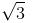)}] = y, then :

Solution:

cos[tan-1(sin(cot-1 (3)½))]
= cos[tan-1(sin(π/6)]
y = cos[tan-1(½)]
Let tan-1 (½) = θ
tanθ = ½
Applying pythagoras, we get hypotenous = (5)½
Cosθ = 2/(5)½
HENCE, y = 2/(5)½

QUESTION: 6

The value of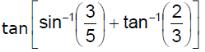is

Solution:
QUESTION: 7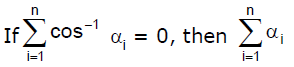Solution:

cos-1 α1 + cos-1 α2 + cos-1 α3 +..........cos-1αn = 0….(1)
As it is given α(i) = 0
From eq(1), α1 = cos0
α1 = 1, α2 = 1…………………… αn = 1
α1 + α2 + α3 +................+ αn
⇒ 1 + 1 + 1 +..................+ n times
⇒ n

QUESTION: 8

The value of x for which sin [cot–1(1+x)] = cos(tan–1x)

Solution:

cos(-1)(x+1)) = cos(tan-1x)
π/2 - cot(-1)(x+1) = 2nπ +- tan-1 x
Put n = 0
=> π/2 - cot(-1)(x+1) +- tan-1 x
= +- tan-1 x
=> π/2 =  tan-1 (+-x) + cot(-1)(x+1)
x+1 = +- x
=> 2x +1 = 0
x = -½

QUESTION: 9

If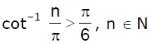, then the maximum value of 'n' is

Solution:

cot−1 n/π > π/6
n/π < cot(π/6)[as cot−1x is a decreasing function]
n < π×(3)1/2
​n < π/3
n < 5.16
so max value of n is 5

QUESTION: 10

The value of sin–1 (sin 12) + cos–1 (cos 12) is equal to

Solution:

sin-1(sin12)+cos-1(cos12)
Here, we have to convert sin12 into a value from -π/2 to π/2
and cos12 into a value from 0 to π.
We can write,
sin-1(sin12)+cos-1(cos12)=sin-1(sin(12-4π))+cos-1(cos(4π-12))
=12-4π+4π-12=0
∴sin-1(sin12)+cos-1(cos12)=0

QUESTION: 11

The value of sin-1[cos{cos-1 (cos x) + sin-1(sin x)}], where x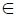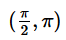Solution:

x implies(pi/2, pi)
= sin-1(cos(cos-1(cosx) + sin-1(sinx)))
= sin-1(cos(x + π - x)
= sin-1(cos π)
= sin-1(-1)
= -π/2

QUESTION: 12

Which of the following is different from 2tan−1x?

Solution:

Explanation : Let α = tan-1 x

x = tanα

∴ cos2α = 2cos2α−1

= 2(1/(1+x2)1/2)2 - 1

= 2 - (1 + x2)/(1+x2) = (1-x2)/(1+x2)

=> 2tan-1

=> 2α = cos-1[(1-x2)/(1+x2)]

Let, tan-1 x = θ

Therefore, tan θ = x

We know that,

tan 2θ = 2tanθ/(1−tan2θ)

tan 2θ = 2x/(1−x2)

sin 2θ = 2tanθ/(1+tan2θ)

sin 2θ = 2x/(1+x2)

QUESTION: 13

If x< 0 then value of tan-1(x) + tan-1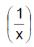is equal to

Solution:

Let y = tan-1 x
x = tan y
⇒ 1/x = 1/tan y
⇒ 1/x = cot y
⇒ 1/x = tan(π/2 - y)
⇒ π/2 - y = tan-1 (1/x)
As we know that y = tan-1 x
π/2 = tan-1(x) + tan-1(1/x)

QUESTION: 14

tan–1 a + tan–1b, where a > 0, b > 0, ab > 1, is equal to

Solution:

tan-1 a + tan-1 b = tan-1[(a+b)/(1 - ab)]    (ab>1)
tan-1[-(a+b)/(1 - ab)]
π - tan-1[(a+b)/(1 - ab)]

QUESTION: 15

If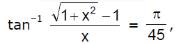then

Solution:
QUESTION: 16

The value of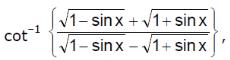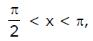is

Solution:
QUESTION: 17

The smallest and the largest values of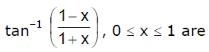Solution:

f(x)=tan−1.(1−x/1+x),0≤x≤1 ltbr gt
Now (1−x/1+x) = 2(1+x)−1
Given 0 ≤ x ≤ 1,
⇒2(1+x)−1∈[0,1]
⇒tan−1.(1−x/1+x)∈[tan−10,tan−11]
=> [0,π4]

QUESTION: 18

If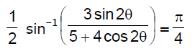, then tan θ is equal to

Solution:

1/2sin−1[3sin2θ/(5+4cos2θ)]=tan−1x
⇒sin−1[3sin2θ/(5+4cos2θ)]=2tan−1x
⇒sin−1[3(2tanθ/(1+tan2θ)/(5+4(1−tan2θ/1+tan2θ))=2tan−1x
⇒sin−1[6tanθ/(5+5tan2θ+4−4tan2θ]=sin1(2x/(1+x2))
⇒sin−1[6tanθ/(9+tan2θ)]=sin1(2x/(1+x2))
⇒sin−1[2(tanθ/3)/1+(tanθ/3)2]=sin1(2x/(1+x2))
⇒x=1/3(tanθ)

QUESTION: 19

The principal value of cos–1 (cos 5) is

Solution:

cos−1(cos5)
=2π−5
as 2π−5 ϵ [0,π]

QUESTION: 20

The value of sin–1 (sin 12) + cos–1 (cos 12) is equal to

Solution:
QUESTION: 21

Which one of the following correct ?

Solution:
QUESTION: 22

The equation sin-1 x - cos-1 x =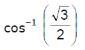has

Solution:

sin-1x - cos-1x = π/6
sin-1x - cos-1x = π/2
Adding both the equations, we get
2sin-1x = 2π/3
sin-1x = π/3
x = sinπ/3  = [(3)½]/2
The equation has unique solution.

QUESTION: 23

The set of values of ‘x’ for which the formula 2 sin-1 x = sin-1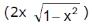is true is

Solution:

π22 ≤ sin−1x ≤ π/2
−π/2 ≤ 2sina ≤ π/2
−π/4 ≤ sina ≤ π4
−1/√2 ≤ a ≤ 1/√2

QUESTION: 24

If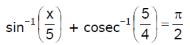, then a value of x is

Solution:
QUESTION: 25

The value of x satisfying sin–1 x + sin–1(1 – x) = cos–1 x are

Solution:

sin−1 x+sin−1(1−x)=cos−1x
⇒ sin−1x + sin−1
(1−x) = π/2−sin−1x
⇒ sin−1
(1−x) = π/2−2sin−1x      ...... (i)
Let sin−1 x = y
⇒ x = siny
Therefore, from (i), we get
sin−1(1−x) = π/2 − 2y
⇒1− x = sin(π / 2 − 2y)
⇒ 1 − x = cos2y
⇒ 1 − x = 1 − 2sin2y
⇒ 1−x = 1 − 2x2
⇒ 2x− x = 0
⇒ x(2x − 1) = 0
⇒ x = 0, 1/2

QUESTION: 26

The number of solutions of the equation tan-1(1 + x) + tan-1(1 – x) = π/2 is

Solution:
QUESTION: 27

The number of solution(s) of the equation Sin-1(1 – x) – 2sin-1 x = π/2 , is / are

Solution: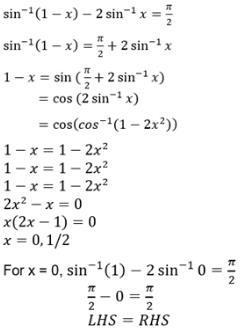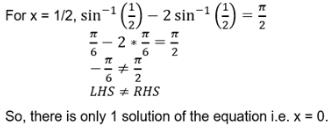QUESTION: 28

The number of solutions of the equation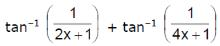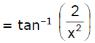is

Solution: